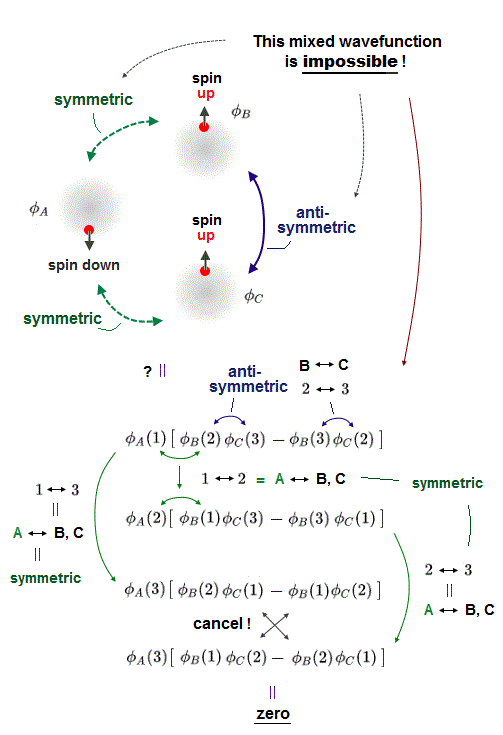﻿ Pauli antisymmetric and molecular attractive wavefunction

# Pauli antisymmetric wavefunction cannot describe molecular bonds.

## Mixing Pauli antisymmetric and molecular bond attractive symmetric wavefunctions is impossible.

(Fig.1) Mixed antisymmetric (= between two same-spin electrons ) and symmetric (= between two opposite-spin electrons ) wavefunctions is impossible.  → Quantum mechanical Pauli principle is wrong.In order to describe actual interactions between molecules, quantum mechanical wavefunctions have to incorporate Pauli repulsion expressed by antisymmetric wavefunction (= between two electrons with the same spin ) and molecular bond attraction expressed by symmetric wavefunctions.

↑ But this mixed antisymmetric and symmetric wavefunctions, which should explain Pauli repulsion and molecular bond attraction at the same time, are intrinsically impossible to make, so quantum mechanical wavefunctions cannot describe actual Pauli repulsion and molecular bond attraction at all.  → Quantum mechanics is wrong.

In the upper figure, φA wavefunction has the down-spin, and the other two φB and φC wavefunctions are supposed to have the up and up spins.

Hence, Pauli repulsion must occur between two electrons with the same up-up spin = two wavefunctions φB (= up-spin ) and φC (= up-spin ) must form Pauli repulsive antisymmetric wavefunctions flipping the sign by exchanging two electrons' variables belonging to φB and φC (= φB ↔ φC ).

Molecular attractive exchange energies must occur between two electrons with the opposite up-down spins = φA (= down-spin ) and the other two φB (= up-spin ), φC (= up-spin ) must form symmetric wavefunctions whose sign don't change under the exchange of two electrons' variables (= φA ↔ φB  or   φA ↔ φC ).

In the upper figure, the 1st equation is antisymmetric wavefunction which flips the sign by exchanging two electrons 2 and 3 (= corresponds to exchanging φB with up-spin and φC with up-spin ).

Exchanging any two electrons existing in two symmetric wavefunctions φA with down-spin and φB (= or φC ) with up-spin must keep the same sign of their wavefunctions.

For example, exchanging two electrons 1 ↔ 3 in the 1st wavefunction means exchanging two electrons of symmetric wavefunctions between φA with down-spin and φB (= or φC ) with up-spin, hence, this symmetric wavefunction should not change its sign by this exchange of two electrons.

But after all, this mixed antisymmetric (= between the same-spin electrons ) and symmetric (= between the opposite-spin electrons ) wavefunction is impossible to make due to cancellation of terms necessary to form symmetric wavefunctions, which become zero, as shown above.

In conclusion, quantum mechanics is wrong, because its Pauli antisymmetric wavefunctions cannot describe Pauli repulsion (= antisymmetric ) and molecular bond attraction (= symmetric wavefunction ) at the same time.2022/5/25 updated. Feel free to link to this site.